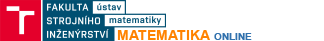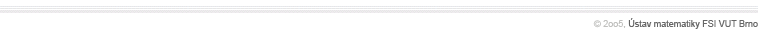Number sequencesDefinition of a sequence, constant sequence, arithmetic sequence, geometric sequence, recurrent seuences-fibonacci sequence, subsequence, bounded sequences, limit of a sequence, calculating limits of sequences, squezing rule, points of condensation, upper and lower limits Sequences defining eProving that (n+1/n)^n converges to eCalculating limits of sequencesStolz theorem, application examples